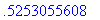As most calculus students know, integration can be a difficult process. Even in Maple, the difficulties have not all been overcome, and symbolic integration should not be considered as completely reliable in all cases. In general it is prudent to check the correctness of integrals, either by comparing definite integrals to their floating-point approximations or by comparing the derivative of an indefinite integral to the integrand.

One common source of trouble is multivalued functions. In particular, a definite integral int(f(x), x = a .. b) may give a wrong answer because the antiderivative is a multivalued function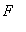with a branch cut crossing the path of integration. Maple does not recognize the branch cut, and returns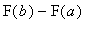as the value of the definite integral. Note that this can happen even when the integrand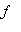is single-valued.

In other cases, whenis multivalued Maple may find an antiderivative for the wrong choice of branch of.

Examples:

The following examples are in Maple V Release 4 and 5. They may be corrected in later releases.

We begin with an antiderivative of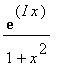.

> F:= int(exp(I*x)/(1+x^2),x);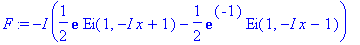This is correct in the sense that differentiation gives us back the integrand (after simplification).

> simplify(diff(F,x));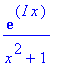Here is a definite integral with this integrand.

> evalf(int(exp(I*x)/(1+x^2), x=-2 .. 2));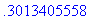This result was obtained by evaluating the antiderivative at the endpoints of the interval.

> evalf(subs(x=2,F) - subs(x=-2,F));However, the answer is wrong. Here is the correct value, obtained by numerical integration.

> evalf(Int(exp(I*x)/(1+x^2), x=-2 .. 2));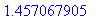> error:= " - "";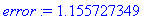The cause of the error is a discontinuity of the antiderivative at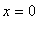. It has a jump of size equal to the error.

> evalf(Limit(F,x=0,left)-Limit(F,x=0,right));The function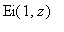which occurs in the antiderivative has a branch cut along the negative real axis. Thus at,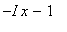hits the branch cut and the term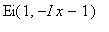in the antiderivative takes a jump.

The next example features a problem with branches of a fractional power.

> f:=((2-3*x)^(-2)*x^(-8))^(1/5);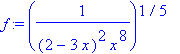> F:= int(f,x);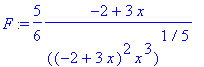> simplify(diff(F,x));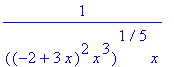This is the same asfor positive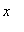, but not for negative(with the principal branch of the fifth root, which Maple uses).

> evalf(subs(x=-1,diff(F,x)));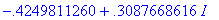> evalf(subs(x=-1,f));